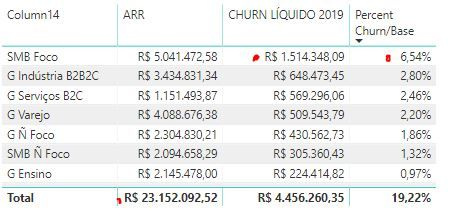cancel
Showing results for
Did you mean:Regular Visitor

## Groups data's percentage

Hello,

I'd like to get a percentage of canceled contracts per segment. I have the total contracts values and the canceled contracts during 2019, but I am not getting the results that I need, for example:Let's think about the item "SMB Foco", I'd like to get the percentage of R\$ 1.514.3483,09 divided per R\$ 5.041.472,58, but when I made a measure to divide it the measure returns the division of R\$ 1.514.348,09 divided per R\$ 23.152.092,52 that is the total of contracts values.

How can I get the division of the group and not of the total?Community Champion

its difficult to advice without example of data model but it seems like you are not use row context. maybe you have an excessive ALL filtration

it should looks like

``````= divide(
calculate(sum('Table'[CHURN LIQUIDO 2019]));
calculate(sum('Table'[ARR]))
)``````

do not hesitate to give a kudo to useful posts and mark solutions as solution

do not hesitate to give a kudo to useful posts and mark solutions as solution